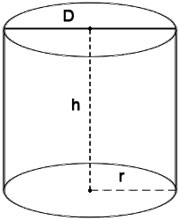# Cylinder Calculator

The volume of a cylinder, as well as the dimensions related to it, can be calculated by inputting the known values into the calculator.

### The calculator includes the following algorithms:

Top Or Bottom Surface Area of a Cylinder: T = B = π*r2

Lateral Surface Area of a Cylinder: L = 2*π*r*h

Total Surface Area of a Cylinder: A = 2*π*r2 + 2*π*r*h = 2*π*r*(r + h)

Volume of a Cylinder: V = π*r2*h

Where π is the constant (3.141592654)

Circular Cylinder CalculatorIt's important to recall the dimensions associated with a cylinder, starting with the definition of a cylinder as a geometrical shape with straight sides parallel to each other and an oval or circular cross-section. The dimensions include diameter, radius, circumference, height, surface area, and volume. The diameter is a straight line passing through the center of a circular or oval shape from one side to another. In the case of a cylinder, the diameter can be found anywhere in its cross-section because of its oval form. The radius is a straight line from the center to any point on the circumference. The circumference is the boundary of a circular or oval shape.

Note that surface area and volume are calculated in square units and cubic units, respectively.